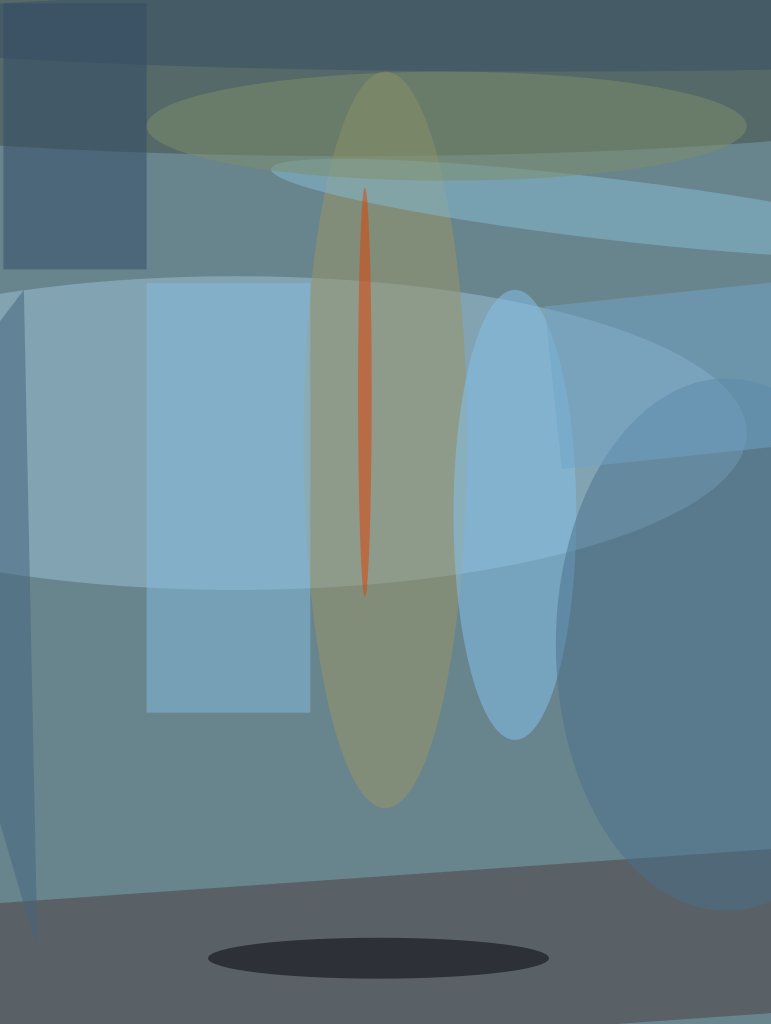The simulation of high-pressure turbulent flows, where the pressure, p, is larger than the critical value, pc, for the species under consideration, is relevant to a wide array of propulsion systems, e.g. gas turbine, diesel, and liquid rocket engines. Most turbulence models, however, have been developed for atmospheric-p turbulent flows. The difference between atmospheric-p and supercritical-p turbulence is that, in the former situation, the coupling between dynamics and thermodynamics is moderate to negligible, but for the latter it is very significant, and can dominate the flow characteristics. The reason for this stems from the mathematical form of the equation of state (EOS), which is the perfect-gas EOS in the former case, and the real-gas EOS in the latter case.

For flows at supercritical pressure, p, the large eddy simulation (LES) equations consist of the differential conservation equations coupled with a real-gas EOS. The equations use transport properties that depend on the thermodynamic variables. Compared to previous LES models, the differential equations contain not only the subgrid scale (SGS) fluxes, but also new SGS terms, each denoted as a “correction.” These additional terms, typically assumed null for atmospheric pressure flows, stem from filtering the differential governing equations, and represent differences between a filtered term and the same term computed as a function of the filtered flow field. In particular, the energy equation contains a heat-flux correction (q-correction) that is the difference between the filtered divergence of the heat flux and the divergence of the heat flux computed as a function of the filtered flow field. In a previous study, there was only partial success in modeling the q-correction term, but in this innovation, success has been achieved by using a different modeling approach.

This analysis, based on a temporal mixing layer Direct Numerical Simulation database, shows that the focus in modeling the q-correction should be on reconstructing the primitive variable gradients rather than their coefficients, and proposes the approximate deconvolution model (ADM) as an effective means of flow field reconstruction for LES heat flux calculation. Further, results for a study conducted for temporal mixing layers initially containing oxygen in the lower stream, and hydrogen or helium in the upper stream, show that, for any LES, including SGS-flux models (constant-coefficient Gradient or Scale-Similarity models, dynamic-coefficient Smagorinsky/Yoshizawa or mixed Smagorinsky/Yoshizawa/Gradient models), the inclusion of the q-correction in the LES leads to the theoretical maximum reduction of the SGS heat-flux difference. The remaining error in modeling this new subgrid term is thus irreducible.

This work was done by Josette Bellan and Ezgi Taskinoglu of Caltech for NASA’s Jet Propulsion Laboratory. For more information, contact This email address is being protected from spambots. You need JavaScript enabled to view it.. NPO-47645##### NASA Tech Briefs Magazine

This article first appeared in the October, 2012 issue of NASA Tech Briefs Magazine.

Read more articles from this issue here.

Read more articles from the archives here.